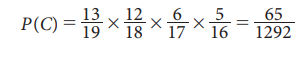Home | | Statistics 11th std | Multiplication Theorem on Probability

# Multiplication Theorem on Probability

Theorem: (Multiplication Theorem of Probability)

Multiplication Theorem on Probability

Theorem 8.5: (Multiplication Theorem of Probability)

If A and B are any two events of an experiment, then### Proof:### Example 8.18

A box contains 7 red and 3 white marbles. Three marbles are drawn from the box one after the other without replacement. Find the probability of drawing three marbles in the alternate colours with the first marble being red.

### Solution:

The event of interest is drawing the marbles in alternate colours with the first is red. This event can occur only when the marbles are drawn in the order (Red , White , Red)

If A and C represent the events of drawing red marbles respectively in the first and the third draws and B is the event of drawing white marble in the second draw, then the required event is AŌł®B Ōł®C. The probability for the occurrence of A Ōł®B Ōł®C can be calculated applying

P(AŌł®BŌł®C) = P (A) P (B/A) P (C/AŌł® B)

Since there are 7 red and 3 white marbles in the box for the first draw,

P(A) = 7/10

Now, there will be 6 red and 3 white marbles in the box for the second draw if the event A has occurred. Hence,

P(B/A) = 3/9

Similarly, there will be 6 red and 2 white marbles in the box for the third draw if the events A and B have occurred. Hence,is the required probability of drawing three marbles in the alternate colours with the first marble being red.

### Example 8.19

Three cards are drawn successively from a well-shuffled pack of 52 playing cards. Find the probability all three cards drawn successively is ace without replacing the card after each draw.

### Solution:

Let A: all the three cards drawn are aces

At the first draw, there will be 4 aces among 52 cards. Having drawn an ace in the first draw, there will be 3 aces among 51 cards. Similarly, there will be 2 aces among 51 cards for the third draw.

Then, as discussed in Example 8.20, by Theorem 8.5### Example 8.20

There are 13 boys and 6 girls in a class. Four students are selected randomly one after another from that class. Find the probability that: (i) all are girls, (ii) first two are boys and next are girls

### Solution:

i. Suppose that

B: all the randomly selected students are girls

There will be 6 girls among 19 students, in total, while selecting the first student; there will be 5 girls among 18 students, in total, while selecting the second student; 4 girls among 17 students, in total, while selecting the third student; and 3 girls among the remaining 16 students, in total, while selecting the fourth student.

Then, by applying the Theorem-8.5 for simultaneous occurrence of these four events, it follows thatii. Suppose that

C: In the randomly selected students the first two are boys and the next are girls

There will be 13 boys among the 19 students, in total, while selecting the first student; there will be 12 boys among 18 students, in total, while selecting the second student; 6 girls among 17 students, in total, while selecting the third student; and 5 girls among the remaining 16 students, in total, while selecting the fourth student.

Then, by applying the Theorem 8.5 for simultaneous occurrence of these four events, it follows thatTags : Theorems, Proof, Solved Example Problems , 11th Statistics : Chapter 8 : Elementary Probability Theory
Study Material, Lecturing Notes, Assignment, Reference, Wiki description explanation, brief detail
11th Statistics : Chapter 8 : Elementary Probability Theory : Multiplication Theorem on Probability | Theorems, Proof, Solved Example Problems